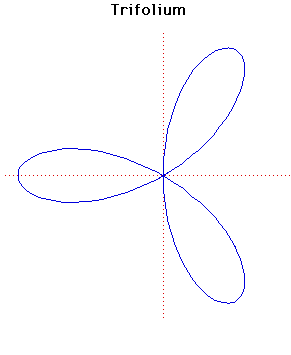# Curves

### TrifoliumCartesian equation:
$(x^{2} + y^{2})(y^{2} + x(x + a)) = 4axy^{2}$
Polar equation:
$r = a \cos \theta (4\sin^{2} \theta - 1)$

### Description

The general form of the folium is given by the formula
$(x^{2} + y^{2})(y^{2} + x(x + b)) = 4axy^{2}$
or, in polar coordinates
$r = -b \cos\theta + 4a \cos\theta \sin^{2}\theta$.
The word folium means 'leaf-shaped'.

There are three special forms of the folium, the simple folium, the double folium and the trifolium. These correspond to the cases
$b = 4a, b = 0, b = a$
respectively in the formula for the general form.

The graph plotted above is the trifolium. There are separate entries for the simple folium and the double folium.

### Associated Curves

Definitions of the Associated curves